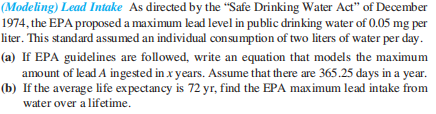### Still have math questions?

Algebra
Question(Modeling) Lead Intake As directed by the "Safe Drinking Water Act" of December 1974, the EPA proposed a maximum lead level in public drinking water of $$0.05 mg$$ per liter. This standard assumed an individual consumption of two liters of water per day.

(a) If EPA guidelines are followed, write an equation that models the maximum amount of lead $$A$$ ingested in $$x$$ years. Assume that there are $$365.25$$ days in a year.

(b) If the average life expectancy is $$72 yr$$ , find the EPA maximum lead intake from water over a lifetime.

$$\left. \begin{array} { l l } { \text { (a) } A = 36.525 x } & { \text { (b) } 2629.8 mg } \end{array} \right.$$